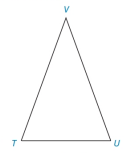Chapter 3.3, Problem 8E### Elementary Geometry for College St...

6th Edition
Daniel C. Alexander + 1 other
ISBN: 9781285195698

#### Solutions

Chapter
Section### Elementary Geometry for College St...

6th Edition
Daniel C. Alexander + 1 other
ISBN: 9781285195698
Textbook Problem
1 views

# For Exercises 1 to 8, use the accompanying drawing. If V U ¯ ≅ V T ¯ and m ∠ V = 40 ° , find m ∠ T .To determine

To find:

The value of mT.

Explanation

Procedure used:

(1) If two sides of a triangle are congruent then angles corresponding to the sides are also congruent to each other.

(2) The sum of measure of angles of a triangle is equal to 180°.

Given:

The given triangle is,

Figure (1)

And,

VU¯VT¯, mV=40°.

Calculation:

Consider the triangle with congruent sides VU¯ and VT¯.

Figure (2)

Equate sum of measure of angles of triangle to 180°

### Still sussing out bartleby?

Check out a sample textbook solution.

See a sample solution

#### The Solution to Your Study Problems

Bartleby provides explanations to thousands of textbook problems written by our experts, many with advanced degrees!

Get Started

#### Find more solutions based on key concepts